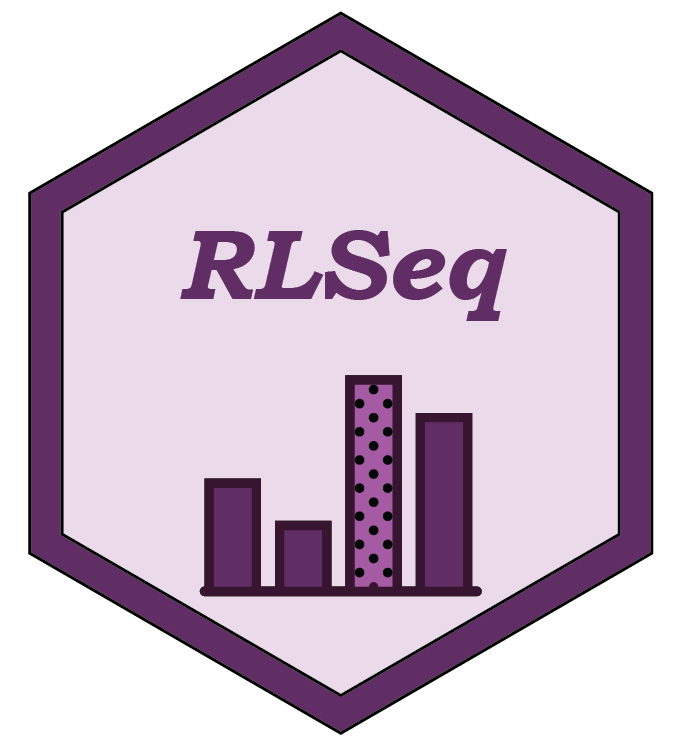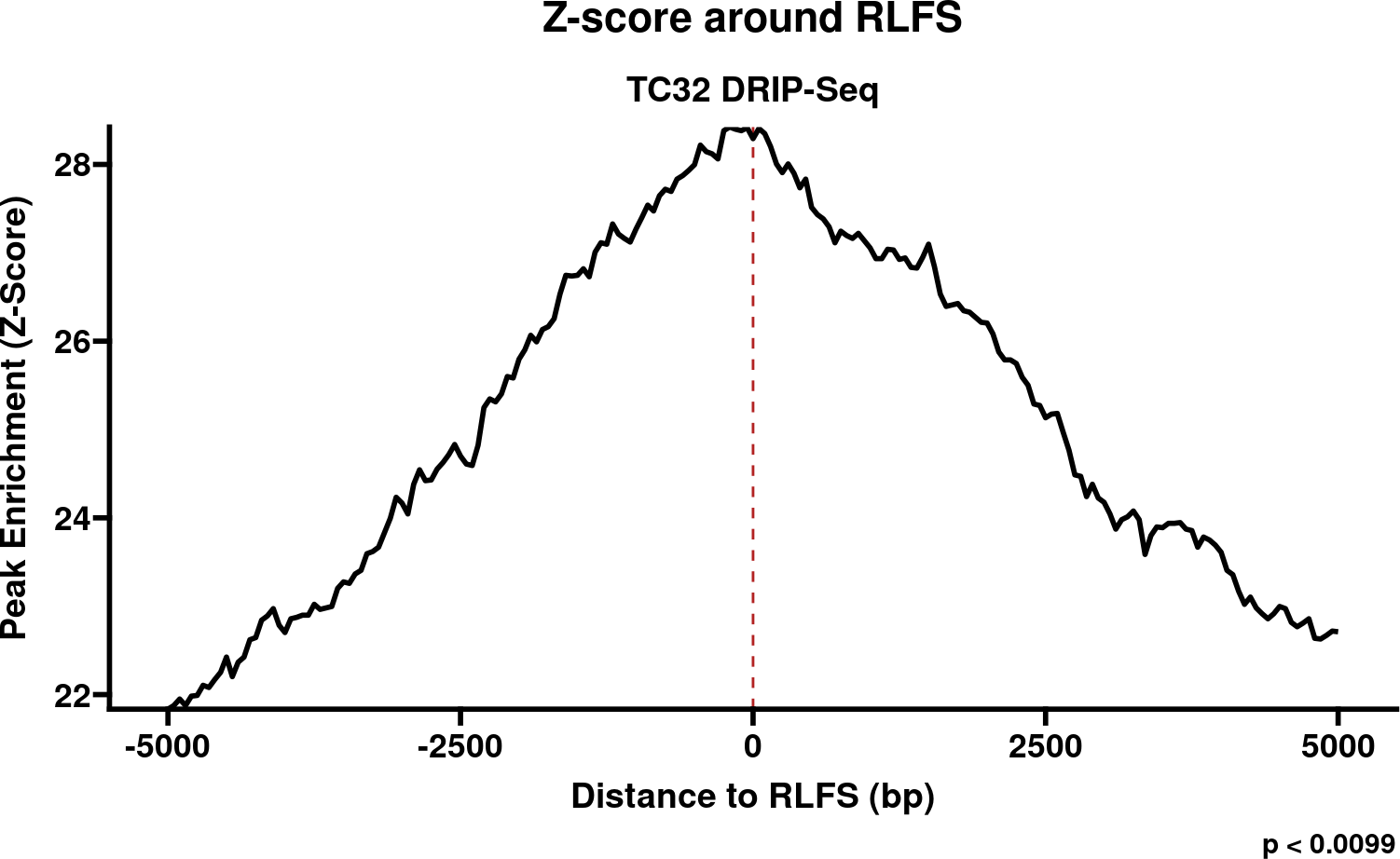# 1 IntroductionRLSeq is a package for analyzing R-loop mapping data sets, and it is a core component of the RLSuite toolchain. It serves two primary purposes: (1) to facilitate the evaluation of data quality, and (2) to enable R-loop data analysis in the context of genomic annotations and the public data sets in RLBase. The main analysis steps can be conveniently run using the `RLSeq()` function. Then, an HTML report can be generated using the `report()` function. Individual steps of this pipeline are also accessible through separate functions which provide custom analysis capabilities.

This vignette will showcase the primary functionality of RLSeq with data from a publicly-available R-loop data mapping study in Ewing sarcoma cell lines, GSE68845. We have selected two DNA-RNA Immunoprecipitation sequencing (DRIP-seq) samples for demonstration purposes: (1) SRX1025890, a positive R-loop mapping sample (“POS”; condition: S9.6 -RNaseH1), and (2) SRX1025892, a negative control (“NEG”; condition S9.6 +RNaseH1). We will begin by showing a quick-start analysis on SRX1025890, and then we will proceed to discuss, in detail, the specific steps of this analysis with both samples.

# 2 Quick-start

Here, we demonstrate a simple analysis workflow which utilizes a publicly-available data set stored in RLBase (a database of R-loop consensus regions and R-loop-mapping experiments, also part of RLSuite). The commands below download these data, run `RLSeq()`, and generate the HTML report.

``````# Peaks and coverage can be found in RLBase
rlbase <- "https://rlbase-data.s3.amazonaws.com"
cvg <- file.path(rlbase, "coverage", "SRX1025890_hg38.bw")

# Initialize data in the RLRanges object.
# Metadata is optional, but improves the interpretability of results
rlr <- RLRanges(
peaks = pks,
coverage = cvg,
genome = "hg38",
mode = "DRIP",
label = "POS",
sampleName = "TC32 DRIP-Seq"
)

# The RLSeq command performs all analyses
rlr <- RLSeq(rlr)

# Generate an html report
report(rlr, reportPath = "rlseq_report_example.html")``````

The report generated by this code is found here.

# 3 Preliminary

## 3.1 Installation

``````if (!requireNamespace("BiocManager", quietly = TRUE))
install.packages("BiocManager")

BiocManager::install("RLSeq")``````

Both packages can also be installed from github.

``````library(remotes)
install_github("Bishop-Laboratory/RLHub")
install_github("Bishop-Laboratory/RLSeq")``````

## 3.2 Obtaining data

RLSeq is compatible with R-loop data generated from a variety of pipelines and tools. However, it is strongly recommended that you use RLPipes, a snakemake-based CLI pipeline tool built specifically for upstream processing of R-loop datasets.

RLPipes can be installed using mamba or conda (slower).

``````# conda install -c conda-forge mamba
mamba create -n rlpipes -c bioconda -c conda-forge rlpipes
conda activate rlpipes``````

A typical config file (`CSV`) should be written as such:

experiment
SRX1025890
SRX1025892

And then the pipeline can be run.

``````RLPipes build -m DRIP rseq_out/ tests/test_data/samples.csv
RLPipes run rseq_out/``````

The resulting directory will contain `peaks/`, `coverage/`, `bam/`, and other processed data sets which are used in downstream analysis.

Note: If you choose to use a different pipeline, use macs2/macs3 for peak calling to ensure compatibility with RLBase.

# 4 End-to-end RLSeq

Here, we describe each step of the analysis pipeline which is run as part of the `RLSeq()` command.

``````library(RLSeq)
library(dplyr)
set.seed(1)``````

## 4.1 Data sets

For this example, we will be using DRIP-Seq data from a 2018 Nature paper on R-loops in Ewing sarcoma (Gorthi et al. 2018). The sample has been IP’d for R-loops (S9.6 -RNaseH1; label: “POS”). The data was processed using RLPipes and uploaded to RLBase. Peaks are converted to `GRanges` objects using a helper function from `regioneR`. URLs and file paths for peak files can also be supplied directly to without this step.

``````rlbase <- "https://rlbase-data.s3.amazonaws.com"

# Get peaks and coverage
s96Cvg <- file.path(rlbase, "coverage", "SRX1025890_hg38.bw")``````

For demonstration purposes, only 10000 ranges are analyzed here.

``````# For expediency, peaks we filter and down-sampled to the top 10000 by padj (V9)
# This is not necessary as part of the typical workflow, however
s96Pks <- s96Pks[s96Pks\$V9 > 2,]
s96Pks <- s96Pks[sample(names(s96Pks), 10000)]``````

Finally, `RLRanges` objects were constructed. These are the primary objects used in all `RLSeq` functions. `RLRanges` are an extension of `GRanges` which provide additional metadata and validation functions.

``````## Build RLRanges ##
# S9.6 -RNaseH1
rlr <- RLRanges(
peaks = s96Pks,
coverage = s96Cvg,
genome = "hg38",
mode = "DRIP",
label = "POS",
sampleName = "TC32 DRIP-Seq"
)``````

## 4.2 Sample quality

Sample quality is assessed by analyzing the association of peaks with R-loop-forming sequences (RLFS). RLFS are genomic sequences that favor the formation of R-loops (Jenjaroenpun et al. 2015). While R-loops can form outside RLFS, there is a strong relationship between them, which provides an unbiased test of whether a set of peaks actually represents successful R-loop mapping.

### 4.2.1 Permutation tests

RLSeq first implements a permutation test to evaluate the enrichment of peaks within RLFS and build a Z-score distribution around RLFS sites.

``````# Analyze RLFS for positive sample
rlr <- analyzeRLFS(rlr, quiet = TRUE)``````

The resulting objects now contain the permutation test results. These results can be easily visualized with the `plotRLFSRes` function.

``````plt <- plotRLFSRes(rlr)
# try() is used to prevent errors on latest MacOS during CI/CD testing.
# You don't need try() when running this normally.
try(plt, silent = TRUE)``````Figure 1: Plot of permutation test results (S9.6 -RNaseH1)

### 4.2.2 Quality classification

The quality classifier is an ensemble model based on an online-learning scheme. It predicts “POS” for samples which are predicted to show robust R-loop mapping and “NEG” for samples which are not. The latest version can be accessed via RLHub. For greater detail, please see the `RLHub::modes` reference. To apply the model and predict sample quality, use the `predictCondition()` function.

``````# Predict
rlr <- predictCondition(rlr)``````

The results from testing our example samples:

``````# Access results
s96_pred <- rlresult(rlr, "predictRes")
cat("Prediction: ", s96_pred\$prediction)``````
``## Prediction:  POS``

## 4.3 Noise analysis

Note: The code in this section does not work on Windows OS machines.

The next step of the RLSeq quality workflow is to analyze the noisiness of the coverage signal for the user-supplied sample (requires that `coverage` is provided when creating the `RLRanges` object). The approach used in this analysis step was derived from the work of Diaz et al., 2012 (Diaz et al. 2012). The method is run using the following function:

``````if (.Platform\$OS.type != "windows") {
rlr <- noiseAnalyze(rlr)
}``````

The results can then be visualized in two ways. A Fingerprint plot and a noiseComparisonPlot.

### 4.3.1 Fingerprint plot

To visualize the results of `noiseAnalyze` we can use a “fingerprint plot” (named after the deepTools implementation by the same name (Ramírez et al. 2016)).

``````if (.Platform\$OS.type != "windows") {
plotFingerprint(rlr)
}``````